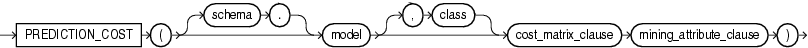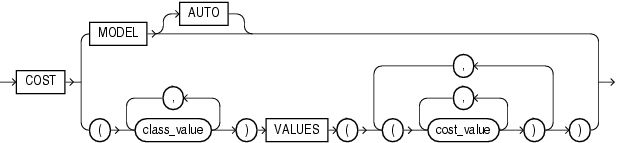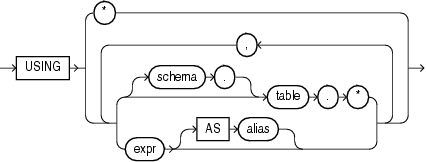# PREDICTION_COST

SyntaxDescription of the illustration prediction_cost.gif

cost_matrix_clause::=Description of the illustration cost_matrix_clause.gif

mining_attribute_clause::=Description of the illustration mining_attribute_clause.gif

Purpose

This function is for use with any classification model created by the `DBMS_DATA_MINING` package or with the Oracle Data Mining Java API. It returns a measure of cost for a given prediction as an Oracle `NUMBER`.

If you specify the optional `class` parameter, then the function returns the cost for the specified class. If you omit the `class` parameter, then the function returns the cost associated with the best prediction. You can use this form in conjunction with the `PREDICTION` function to obtain the best pair of prediction value and cost.

The `COST` clause is relevant for all classification models.

• Specify `COST` `MODEL` to indicate that the scoring should be performed by taking into account the scoring cost matrix associated with the model. If no such scoring cost matrix exists, then the database returns an error.

• Specify `COST` `MODEL` `AUTO` if the existence of a cost matrix is unknown. In this case the function returns the cost using the stored cost matrix if it exists. If no stored cost matrix exists, then the function applies the unit cost matrix (0's on the diagonal and 1's everywhere else). This is equivalent to one minus probability for the given class..

• Use the `VALUES` clause (the bottom branch of the `cost_matrix_clause`) to specify an inline cost matrix. You can use an inline cost matrix regardless of whether the model has an associated scoring cost matrix.

The `mining_attribute_clause` behaves as described for the `PREDICTION` function. Refer to mining_attribute_clause.

Example

The following example finds the ten customers living in Italy who are least expensive to convince to use an affinity card.

This example and the prerequisite data mining operations can be found in the demo file `\$ORACLE_HOME/rdbms/demo/dmdtdemo.sql`. General information on data mining demo files is available in Oracle Data Mining Administrator's Guide. The example is presented here to illustrate the syntactic use of the function.

```WITH
cust_italy AS (
SELECT cust_id
FROM mining_data_apply_v
WHERE country_name = 'Italy'
ORDER BY PREDICTION_COST(DT_SH_Clas_sample, 1 COST MODEL USING *) ASC, 1
)
SELECT cust_id
FROM cust_italy
WHERE rownum < 11;

CUST_ID
----------
100081
100179
100185
100324
100344
100554
100662
100733
101250
101306

10 rows selected.
```